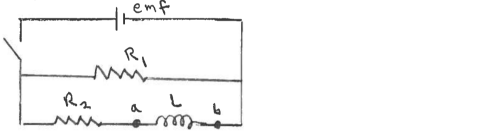# Problem: Consider the circuit shown in the sketch. The battery emf is 48.0 V,  R1 = 16.0 Ω, and R2 = 6.00 Ω. Initially no currents are flowing. Then the switch is closed. After the switch has been closed a long time, what is the voltage  vab across the inductor?

###### FREE Expert Solution
87% (123 ratings)
###### Problem Details

Consider the circuit shown in the sketch. The battery emf is 48.0 V,  R1 = 16.0 Ω, and R2 = 6.00 Ω. Initially no currents are flowing. Then the switch is closed. After the switch has been closed a long time, what is the voltage  vab across the inductor?Frequently Asked Questions

What scientific concept do you need to know in order to solve this problem?

Our tutors have indicated that to solve this problem you will need to apply the LR Circuits concept. You can view video lessons to learn LR Circuits. Or if you need more LR Circuits practice, you can also practice LR Circuits practice problems.

How long does this problem take to solve?

Our expert Physics tutor, Juan took 2 minutes and 11 seconds to solve this problem. You can follow their steps in the video explanation above.